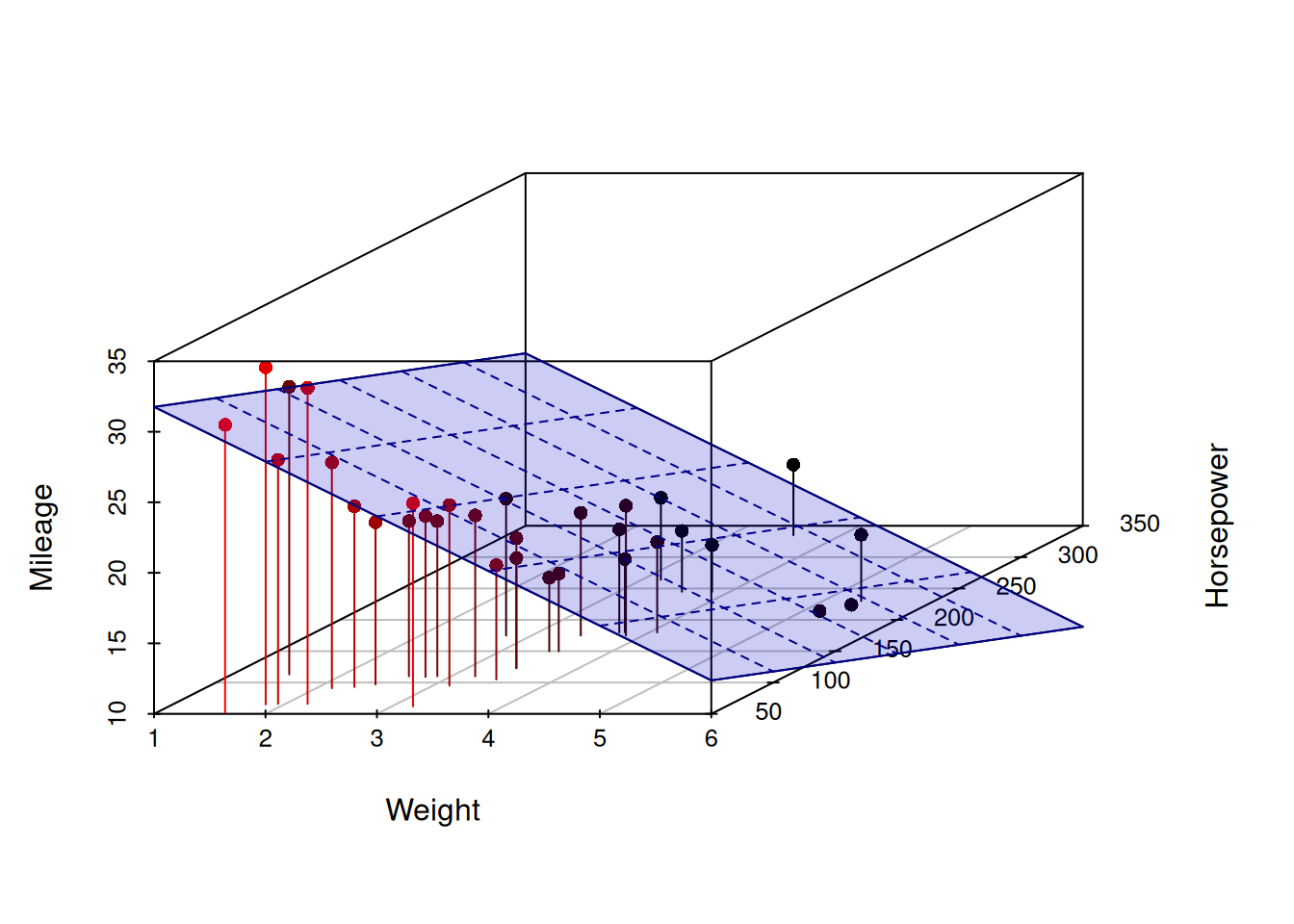This book is in Open Review. I want your feedback to make the book better for you and other readers. To add your annotation, select some text and then click the on the pop-up menu. To see the annotations of others, click the button in the upper right hand corner of the page

# Chapter 11 Multiple Linear Regression

While simple linear regression provides a basic understanding of the idea of capturing the relations between variables, it is obvious that in reality there are more than one external variable that would impact the response variable. This means that instead of (10.1) we should have: $\begin{equation} y_j = \beta_0 + \beta_1 x_{1,j} + \beta_2 x_{2,j} + \dots + \beta_{k-1} x_{k-1,j} + \epsilon_j , \tag{11.1} \end{equation}$ where $$\beta_i$$ is a $$i$$-th parameter for the respective $$i$$-th explanatory variable and there is $$k-1$$ of them in the model, meaning that when we want to estimate this model, we will have $$k$$ unknown parameters. The regression line of this model in population (aka expectation conditional on the values of explanatory variables) is: $\begin{equation} \mu_{y,j} = \mathrm{E}(y_j | \mathbf{x}_j) = \beta_0 + \beta_1 x_{1,j} + \beta_2 x_{2,j} + \dots + \beta_{k-1} x_{k-1,j} , \tag{11.2} \end{equation}$ while in case of a sample estimation of the model we will use: $\begin{equation} \hat{y}_j = b_0 + b_1 x_{1,j} + b_2 x_{2,j} + \dots + b_{k-1} x_{k-1,j} . \tag{11.3} \end{equation}$ While the simple linear regression can be represented as a line on the plane with an explanatory variable and a response variable, the multiple linear regression cannot be easily represented in the same way. In case of two explanatory variables the plot becomes three dimensional and the regression line transforms into regression plane.Figure 11.1: 3D scatterplot of Mileage vs Weight of a car and its Engine Horsepower.

Figure 11.1 demonstrates a three dimensional scatterplot with the regression plane, going through the points, similar to how the regression line went through the two dimensional scatterplot 10.1. These sorts of plots are already difficult to read, but the situation becomes even more challenging, when more than two explanatory variables are under consideration: plotting 4D, 5D etc is not a trivial task. Still, what can be said about the parameters of the model even if we cannot plot it in the same way, is that they represent slopes for each variable, in a similar manner as $$\beta_1$$ did in the simple linear regression.